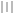# Mechanical vibration (2)

## 振动的能量（弹簧振子）

• 公式： $E=E_{K}+E_{p}=\frac{1}{2}kA^{2}$

• $E_{k}=\frac{1}{2}kA^{2}sin^{2}(\omega t + \varphi)$

• $E_{p}=\frac{1}{2}kA^{2}cos^{2}(\omega t + \varphi)$

• 单位：J

• 特点：

• 振子在振动过程中，动能和势能分别随时间变化，但任一时刻总机械能保持不变。

• 动能和势能的变化频率是弹簧振子振动频率的两倍。

• 频率一定时，谐振动的总能量与振幅的平方成正比。（适合于任何谐振系统）

## 振动的能量（单摆）

• 公式： $E=E_{k}+E_{p}=mgh$ （ $h$ 是速度为0时，摆锤距离零势能面的高度）
• 单位：J
• 判断一个振动是否是简谐运动
1. 看是否满足 $F=kx$ ，回复力与距离平衡点的距离成正比。这个是简谐运动的定义。所以拿这个来证明是肯定没错的。进一步也可以拿加速度与距离来做一个判断
2. 看是否满足简谐运动的运动方程 $x=Acos(\omega t + \varphi)$
3. 如果是弹簧振子还可以看，振动的能量是否正比于振幅的平方Invitation
USTC-茶糜花开
FeynmanDirac
created:12/03/2022
Welcome to USTC-茶糜花开

This is an identification card as an honored membership of FeynmanDirac

Happy to see you follow FeynmanDirac, enjoy science together验证码启动中...